Balbharti Maharashtra State Board 12th Physics Textbook Solutions Chapter 9 Current Electricity Textbook Exercise Questions and Answers.

## Maharashtra State Board 12th Physics Solutions Chapter 9 Current Electricity

1. Choose the correct option.

i) Kirchhoff’s first law, i.e., ΣI = 0 at a junction, deals with the conservation of
(A) charge
(B) energy
(C) momentum
(D) mass
(A) charge

ii) When the balance point is obtained in the potentiometer, a current is drawn from
(A) both the cells and auxiliary battery
(B) cell only
(C) auxiliary battery only
(D) neither cell nor auxiliary battery
(D) neither cell nor auxiliary batteryiii) In the following circuit diagram, an infinite series of resistances is shown. Equivalent resistance between points A and B is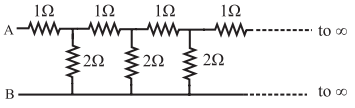(A) infinite
(B) zero
(C) 2 Ω
(D) 1.5 Ω
(C) 2 Ω

iv) Four resistances 10 Ω, 10 Ω, 10 Ω and 15 Ω form a Wheatstone’s network. What shunt is required across 15 Ω resistor to balance the bridge
(A) 10 Ω
(B) 15 Ω
(C) 20 Ω
(D) 30 Ω
(D) 30 Ω

v) A circular loop has a resistance of 40 Ω. Two points P and Q of the loop, which are one quarter of the circumference apart are connected to a 24 V battery, having an internal resistance of 0.5 Ω. What is the current flowing through the battery.
(A) 0.5 A
(B) 1A
(C) 2A
(D) 3A
(D) 3Avi) To find the resistance of a gold bangle, two diametrically opposite points of the bangle are connected to the two terminals of the left gap of a metre bridge. A resistance of 4 Ω is introduced in the right gap. What is the resistance of the bangle if the null point is at 20 cm from the left end?
(A) 2 Ω
(B) 4 Ω
(C) 8 Ω
(D) 16 Ω
(A) 2 Ω

i) Define or describe a Potentiometer.
The potentiometer is a device used for accurate measurement of potential difference as well as the emf of a cell. It does not draw any current from the circuit at the null point. Thus, it acts as an ideal voltmeter and it can be used to determine the internal resistance of a cell. It consist of a long uniform wire AB of length L, stretched on a wooden board. A cell of stable emf (E), with a plug key K in series, is connected across AB as shown in the following figure.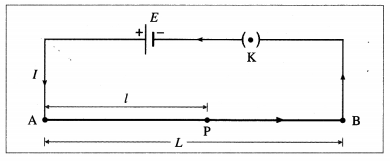Potential gradient is defined as the potential difference (the fall of potential from the high potential end) per unit length of the wire.

iii) Why should not the jockey be slided along the potentiometer wire?
Sliding the jockey on the potentiometer wire decreases the cross sectional area of the wire and thereby affects the fall of potential along the wire. This affects the potentiometer readings. Flence, the jockey should not be slided along the potentiometer wire.

iv) Are Kirchhoff’s laws applicable for both AC and DC currents?
Kirchhoff’s laws are applicable to both AC and DC ’ circuits (networks). For AC circuits with different loads, (e.g. a combination of a resistor and a capacitor, the instantaneous values for current and voltage are considered for addition.

[Note : Gustav Robert Kirchhoff (1824-87), German physicist, devised laws of electrical network and, with Robert Wilhelm Bunsen, (1811-99), German chemist, did pioneering work in chemical spectroscopy. He also con-tributed to radiation.]v) In a Wheatstone’s meter-bridge experiment, the null point is obtained in middle one third portion of wire. Why is it recommended?

1. The value of unknown resistance X, may not be accurate due to non-uniformity of the bridge wire and development of contact resistance at the ends of the wire.
2. To minimise these errors, the value of R is so adjusted that the null point is obtained in the middle one-third of the wire (between 34 cm and 66 cm) so that the percentage errors in the measurement of IX and IR are minimum and nearly the same.

vi) State any two sources of errors in meterbridge experiment. Explain how they can be minimized.
The chief sources of error in the metre bridge experiment are as follows :

1. The bridge wire may not be uniform in cross section. Then the wire will not have a uniform resistance per unit length and hence its resistance will not be proportional to its length.
2. End resistances at the two ends of the wire may be introduced due to
1. the resistance of the metal strips
2. the contact resistance of the bridge wire with the metal strips
3. unmeasured lengths of the wire at the ends because the contact points of the wire with the metal strips do not coincide with the two ends of the metre scale attached.

Such errors are almost unavoidable but can be minimized considerably as follows :

1. Readings must be taken by adjusting the standard known resistance such that the null point is obtained close to the centre of the wire. When several readings are to be taken, the null points should lie in the middle one-third of the wire.
2. The measurements must be repeated with the standard resistance (resistance box) and the unknown resistance interchanged in the gaps of the bridge, obtaining the averages of the two results.

vii) What is potential gradient? How is it measured? Explain.
Consider a potentiometer consisting of a long uniform wire AB of length L and resistance R, stretched on a wooden board and connected in series with a cell of stable emf E and internal resistance r and a plug key K as shown in below figure.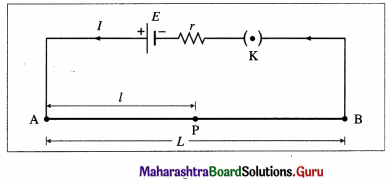Let I be the current flowing through the wire when the circuit is closed.
Current through AB, I = $$\frac{E}{R+r}$$
Potential difference across AB. VAB = IR
∴ VAB = $$\frac{E R}{(R+r)}$$
The potential difference (the fall of potential from the high potential end) per unit length of the wire,
$$\frac{V_{\mathrm{AB}}}{L}=\frac{E R}{(R+r) L}$$
As long as E and r remain constant, $$\frac{V_{\mathrm{AB}}}{L}$$ will remain constant, $$\frac{V_{\mathrm{AB}}}{L}$$ is known as potential gradient along

AB and is denoted by K. Thus the potential gradient is calculated by measuring the potential difference between ends of the potentiometer wire and dividing it by the length of the wire.
Let P be any point on the wire between A and B and AP = l = length of the wire between A and P.
Then VAP = Kl
∴ VAP ∝ l as K is constant in a particular case. Thus, the potential difference across any length of the potentiometer wire is directly proportional to that length. This is the principle of the potentiometer.

viii) On what factors does the potential gradient of the wire depend?
The potential gradient depends upon the potential difference between the ends of the wire and the length of the wire.ix) Why is potentiometer preferred over a voltmeter for measuring emf?
A voltmeter should ideally have an infinite resistance so that it does not draw any current from the circuit. However a voltmeter cannot be designed to have infinite resistance. A potentiometer does not draw any current from the circuit at the null point. Therefore, it gives more accurate measurement. Thus, it acts as an ideal voltmeter.

x) State the uses of a potentiometer.
The applications (uses) of the potentiometer :

1. Voltage divider :
The potentiometer can be used as a voltage divider to change the output voltage of a voltage supply.
2. Audio control:
Sliding potentiometers are commonly used in modern low-power audio systems as audio control devices. Both sliding (faders) and rotary potentiometers (knobs) are regularly used for frequency attenuation, loudness control and for controlling different characteristics of audio signals.
3. Potentiometer as a sensor:
If the slider of the potentiometer is connected to the moving part of a machine, it can work as a motion sensor. A small displacement of the moving part causes a change in potential which is further amplified using an amplifier circuit. The potential difference is calibrated in terms of displacement of the moving part.
4. To measure the emf (for this, the emf of the standard cell and potential gradient must be known).
5. To compare the emf’s of two cells.
6. To determine the internal resistance of a cell.

xi) What are the disadvantages of a potentiometer?
Disadvantages of a potentiometer over a voltmeter :

1. The use of a potentiometer is an indirect measurement method while a voltmeter is a direct reading instrument.
2. A potentiometer is unwieldy while a voltmeter is portable.
3. Unlike a voltmeter, the use of a potentiometer in measuring an unknown emf requires a standard . source of emf and calibration.

xii) Distinguish between a potentiometer and a voltmeter.

 Potentiometer Voltmeter 1. A potentiometer is used to determine the emf of a cell, potential difference and internal resistance. 1. A voltmeter can be used to measure the potential difference and terminal voltage of a cell. But it cannot be used to measure the emf of a cell. 2. Its accuracy and sensitivity are very high. 2. Its accuracy and sensitivity are less as compared to a potentiometer. 3. It is not a portable instrument. 3. It is a portable instrument. 4. It does not give a direct reading. 4. It gives a direct reading.

xiii) What will be the effect on the position of zero deflection if only the current flowing through the potentiometer wire is
(i) increased (ii) decreased.
(1) On increasing the current through the potentiometer wire, the potential gradient along the wire will increase. Hence, the position of zero deflection will occur at a shorter length.

(2) On decreasing the current through the potentiometer wire, the potential gradient along the wire will decrease. Hence, the position of zero deflection will occur at a longer length.
[Note : In the usual notation,
E1 = ($$\frac{I R}{L}$$) l1 = constant
Hence, (i) E, decreases when I is increased (ii) l1 increases when I is decreased.]Question 3.
Obtain the balancing condition in case of a Wheatstone’s network.
Wheatstone’s network or bridge is a circuit for indirect measurement of resistance by null com-parison method by comparing it with a standard known resistance. It consists of four resistors with resistances P, Q, R and S arranged in the form of a quadrilateral ABCD. A cell (E) with a plug key (K) in series is connected across one diagonal AC and a galvanometer (G) across the other diagonal BD as shown in the following figure.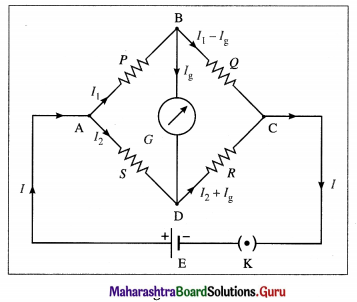With the key K dosed, currents pass through the resistors and the galvanometer. One or more of the resistances is adjusted until no deflection in the galvanometer can be detected. The bridge is then said to be balanced.

Let I be the current drawn from the cell. At junction A, it divides into a current I1 through P and a current I2 through S.
I = I1 + I2 (by Kirchhoff’s first law).
At junction B, current Ig flows through the galvanometer and current I1 – Ig flows through Q. At junction D, I2 and Ig combine. Hence, current I2 + Ig flows through R from D to C. At junction C, I1 – Ig and I2 + Ig combine. Hence, current I1 + I2(= I) leaves junction C.

Applying Kirchhoff’s voltage law to loop ABDA in a clockwise sense, we get,
– I1P – IgG + I2S = 0 …………… (1)
where G is the resistance of the galvanometer.
Applying Kirchhoff’s voltage law to loop BCDB in a clockwise sense, we get,
– (I1 – Ig)Q + (I2 + Ig)R + IgG = 0 ………….. (2)
When Ig = 0, the bridge (network) is said to be balanced. In that case, from Eqs. (1) and (2), we get,
I1P = I2S …………… (3)
and I1Q = I2R ………….. (4)
From Eqs. (3) and (4), we get,
$$\frac{P}{Q}=\frac{S}{R}$$
This is the condition of balance.
Alternative Method: When no current flows through the galvanometer, points B and D must be at the same potential.
∴ VB = VD
∴ VA – VB = VA – VD …………. (1)
and VB – VC = VD – VC ………… (2)
Now, VA – VB = I1P and VA – VD = I2S ………….. (3)
Also, VB – VC = I1Q and VD – VC = I2R …………. (4)
Substituting Eqs. (3) and (4) in Eqs. (1) and (2), we get,
I1P = I2S . ………… (5)
and I1Q = I2R …(6)
Dividing Eq. (5) by Eq. (6), we get,
$$\frac{P}{Q}=\frac{S}{R}$$
This is the condition of balance.

[ Note : In the determination of an unknown resistance using Wheatstone’s network, the unknown resistance is connected in one arm of the network (say, AB), and a standard known variable resistance is connected in an adjacent arm. Then, the other two arms are called the ratio arms. Also, because the positions of the cell and galvanometer can be interchanged, without a change in the condition of balance, the branches AC and BD in figure are called the conjugate arms. ]

Question 4.
Explain with neat circuit diagram, how you will determine the unknown resistance by using a meter-bridge.
A metre bridge consists of a rectangular wooden board with two L-shaped thick metallic strips fixed along its three edges. A single thick metallic strip separates two L-shaped strips. A wire of length one metre and uniform cross section is stretched on a metre scale fixed on the wooden board. The ends of the wire are fixed to the L-shaped metallic strips.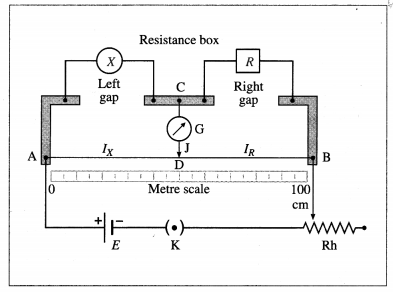An unknown resistance X is connected in the left gap and a resistance box R is connected in the right gap as shown in above figure. One end of a centre-zero galvanometer (G) is connected to terminal C and the other end is connected to a pencil jockey (J). A cell (E) of emf E, plug key (K) and rheostat (Rh) are connected in series between points A and B.

Working : Keeping a suitable resistance (R) in the resistance box, key K is closed to pass a current through the circuit. The jockey is tapped along the wire to locate the equipotential point D when the galvanometer shows zero deflection. The bridge is then balanced and point D is called the null point and the method is called as null deflection method. The distances lX and lR of the null point from the two ends of the wire are measured.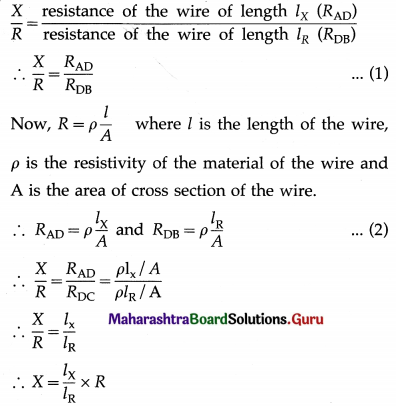As R, lX and lR are known, the unknown resistance X can be calculated.Question 5.
Describe Kelvin’s method to determine the resistance of a galvanometer by using a meter bridge.
Kelvin’s method :
Circuit: The metre bridge circuit for Kelvin’s method of determination of the resistance of a galvanometer is shown in below figure. The galvanometer whose resistance G is to be determined, is connected in one gap of the metre bridge. A resistance box providing a variable known resistance R is connected in the other gap. The junction B of the galvanometer and the resistance box is con-nected directly to a pencil jockey. A cell of emf E, a key (K) and a rheostat (Rh) are connected across AC.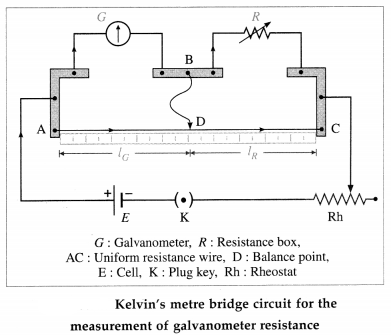Working : Keeping a suitable resistance R in the resistance box and maximum resistance in the rheostat, key K is closed to pass the current. The rheostat resistance is slowly reduced such that the galvanometer shows about 2 / 3rd of the full-scale deflection.

On tapping the jockey at end-points A and C, the galvanometer deflection should change to opposite sides of the initial deflection. Only then will there be a point D on the wire which is equipotential with point B. The jockey is tapped along the wire to locate the equipotential point D when the galvanometer shows no change in deflection. Point D is now called the balance point and Kelvin’s method is thus an equal deflection method. At this balanced condition,
$$\frac{G}{R}=\frac{\text { resistance of the wire of length } l_{G}}{\text { resistance of the wire of length } l_{R}}$$
where IG = the length of the wire opposite to the galvanometer, IR = the length of the wire opposite to the resistance box.
If λ = the resistance per unit length of the wire,
$$\frac{G}{R}=\frac{\lambda l_{G}}{\lambda l_{R}}=\frac{l_{G}}{l_{R}}$$
∴ G = R$$\frac{l_{G}}{l_{R}}$$
The quantities on the right hand side are known, so that G can be calculated.
[Note : The method was devised by William Thom-son (Lord Kelvin, 1824-1907), British physicist.]

Question 6.
Describe how a potentiometer is used to compare the emfs of two cells by connecting the cells individually.
A battery of stable emf E is used to set up a potential gradient V/L along a potentiometer wire, where V = potential difference across the length L of the wire. The positive terminals of the cells, whose emf’s (E1 and E2) are to be compared, are connected to the high potential terminal A. The negative terminals of the cells are connected to a galvanometer G through a two-way key. The other terminal of the galvanometer is connected to a pencil jockey. The emf E should be greater than both the emf’s E1 and E2.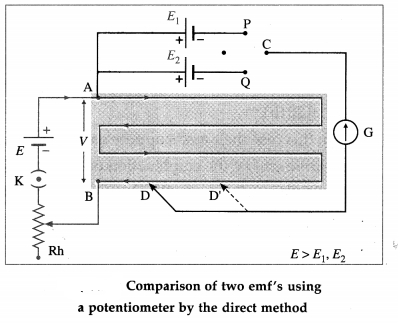Connecting point P to C, the cell with emf E1 is brought into the circuit. The jockey is tapped along the wire to locate the null point D at a distance l1 from A. Then,
E1 = Z1(V/L)
Now, without changing the potential gradient (i.e., without changing the rheostat setting) point Q (instead of P) is connected to C, bringing the cell with emf E2 into the circuit. Let its null point D’ be at a distance l2 from A, so that
E2 = l2(V/L)
∴ $$\frac{E_{1}}{E_{2}}=\frac{l_{1}}{l_{2}}$$
Hence, by measuring the corresponding null lengths l1 and l2, E1/E2 can be calculated. The experiment is repeated for different potential gradients using the rheostat.
[Note : This method is used when the two emf’s have comparable magnitudes. Then, the errors of measurement of their balancing lengths will also be of comparable magnitudes.]Question 7.
Describe how a potentiometer is used to compare the emfs of two cells by combination method.
A battery of stable emf E is used to set up a potential gradient V/L, along the potentiometer wire, where V = potential difference across length L of the wire. The positive terminal of the cell 1 is connected to the higher potential terminal A of the potentiometer; the negative terminal is connected to the galvanometer G through the reversing key. The other terminal of the galvanometer is connected to a pencil jockey. The cell 2 is connected across the remaining two opposite terminals of the reversing key. The other terminal of the galvanometer is connected to a pencil jockey. The emf E1 should be greater than the emf E2; this can be adjusted by trial and error.

Two plugs are inserted in the reversing key in positions 1 – 1. Here, the two cells assist each other so that the net emf is E1 + E2. The jockey is tapped along the wire to locate the null point D. If the null point is a distance l1 from A,
E1 + E2 = l1 (V/L)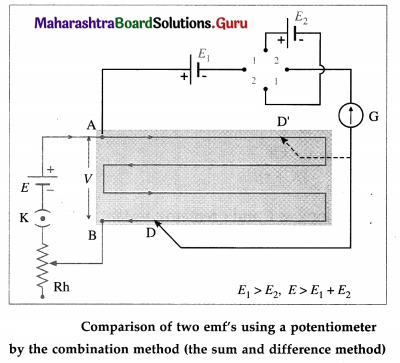For the same potential gradient (without changing the rheostat setting), the plugs are now inserted into position 2 – 2. (instead of 1 – 1). The emf E2 then opposes E1 and the net emf is E1 – E2. The new null point D’ is, say, a distance l2 from A and
E1 – E2 = l2 (V/L)
∴ $$\frac{E_{1}+E_{2}}{E_{1}-E_{2}}=\frac{l_{1}}{l_{2}}$$ ∴ $$\frac{E_{1}}{E_{2}}=\frac{l_{1}-l_{2}}{l_{1}-l_{2}}$$
Here, the emf E should be greater than E1 + E2. The experiment is repeated for different potential gradients using the rheostat.
[Note : This method is used when E1 » E2, so that E1 + E2 and E1 – E2 have comparable magnitudes. Then, the errors of measurement of l1 and l2 will also be of comparable magnitudes.]

Question 8.
Describe with the help of a neat circuit diagram how you will determine the internal resistance of a cell by using a potentiometer. Derive the necessary formula.
Principle : A cell of emf £ and internal resistance r, which is connected to an external resistance R, has its terminal potential difference V less than its emf E. If I is the corresponding current,
$$\frac{E}{V}=\frac{I(R+r)}{I R}=\frac{R+r}{R}$$ = 1 + $$\frac{r}{R}$$ (when R → ∞, V → E)
∴ r = $$\frac{E-V}{V}$$ R
Working : A battery of stable emf E’ is used to set up a potential gradient V/L, along the potentiometer wire, where V = potential difference across the length L of the wire. The negative terminal is connected through a centre-zero galvanometer G to a pencil jockey. A resistance box R with a plug key K in series is connected across the cell.

Firstly, key K is kept open; then, effectively, R = ∞. The jockey is tapped on the potentiometer wire to locate the null point D. Let the null length
E = (VAB/L)l1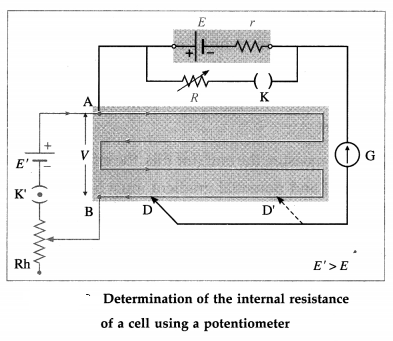With the same potential gradient, and a small resistance R in the resistance box, key K is closed. The new null length AD’ = l2 for the terminal potential difference V is found :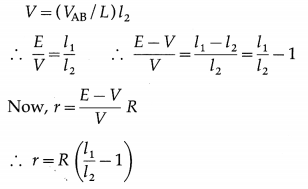R, l1, and l2 being known, r can be calculated. The experiment is repeated with different potential gradients using the rheostat or with different values of R.

Question 9.
On what factors does the internal resistance of a cell depend?
The internal resistance of a cell depends on :

1. Nature of the electrolyte :
The greater the conductivity of the electrolyte, the lower is the internal resistance of the cell.
2. Separation between the electrodes :
The larger the seperation between the electrodes of the cell, the higher is the internal resistance of the cell. This is because the ions have to cover a greater distance before reaching an electrode.
3. Nature of the electrodes.
4. The internal resistance is inversly proportional to the common area of the electrodes dipping in the electrolyte.Question 10.
A battery of emf 4 volt and internal resistance 1 Ω is connected in parallel with
another battery of emf 1 V and internal resistance 1 Ω (with their like poles connected together). The combination is used to send current through an external resistance of 2 Ω. Calculate the current through the external resistance.
Let I1 and I2 be the currents through the two branches as shown in below figure. The current through the 2 Q resistance will be (I1 + I2) [Kirchhoff’s current law].
Applying Kirchhoff’s voltage law to loop ABCDEFA, we get,
-2(I1 + I2) – 1(I1) + 4 = 0
∴ 3I1 + 2I1 = 4 …………… (1)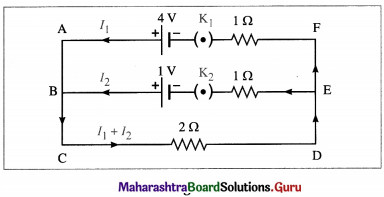Applying Kirchhoff’s voltage law to loop BCDEB, we get,
-2(I1 + I2) – 1(I2) + 1 = 0
2I1 + 3I2 = 1 ………… (2)
Multiplying Eq. (1) by 2 and Eq. (2) by 3, we get,
6I1 + 4I2 = 8 ………….(3)
and 6I1 + 9I2 = 3 ……………. (4)
Subtracting Eq. (4) from Eq. (3), we get,
– 5I2 =5
∴ I2 = -1A
The minus sign shows that the direction of current I2 is opposite to that assumed. Substituting this value of 12 in Eq. (1), we get,
3I1 + 2(-1) = 4
∴3I1 = 4 + 2 = 6
∴ I1 = 2A
Current through the 2 0 resistance = I1 + I2 = 2 – 1
= 1 A. It is in the direction as shown in the figure.
[Note : We may as well consider loop ABEFA and write the corresponding equation. But it does not provide any additional information.]

Question 11.
Two cells of emf 1.5 Volt and 2 Volt having respective internal resistances of 1 Ω and 2 Ω are connected in parallel so as to send current in same direction through an external resistance of 5 Ω. Find the current through the external resistance.
Let I1 and I2 be the currents through the two branches as shown in below figure. The current through the 5 Q resistor will be I1 + I2 [Kirchhoff’s current law].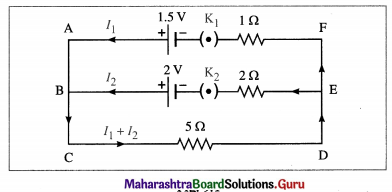Applying Kirchhoff’s voltage law to loop w ABCDEFA, we get,
– 5(I1 + I2) – I1 + 1.5 = 0
∴ 6I1 + 5I2 = 1.5 ……………. (1)
Applying Kirchhoff’s voltage law to loop BCDEB, we get,
– 5(I1 + I2) – 2I2 + 2 = 0
∴ 5I1 + 7I2 = 2 ……………(2)
Multiplying Eq. (1) by 5 and Eq. (2) by 6, we get,
30I1 + 25I2 = 7.5 …………… (3)
and 30I1 + 42I2 = 12 …………. (4)
Subtracting Eq. (3) from Eq. (4), we get,
17I2 = 4.5
∴ I2 = $$\frac{4.5}{17}$$ A
Substituting this value of I2 in Eq. (1), we get,
6I1 + 5($$\frac{4.5}{17}$$) = 1.5
∴ 6I1 + $$\frac{22.5}{17}$$ = 1.5
∴ 6I1 = 1.5 – $$\frac{22.5}{17}$$ = $$\frac{28.5-22.5}{17}$$
= $$\frac{3}{17}$$
∴ I1 = $$\frac{3}{17 \times 6}=\frac{0.5}{17}$$ A
Current through the 5 Q resistance (external resistance)
= I1 + I2 = $$\frac{0.5}{17}+\frac{4.5}{17}=\frac{5}{17}$$ A

Question 12.
A voltmeter has a resistance 30 Ω. What will be its reading, when it is connected across a cell of emf 2 V having internal resistance 10 Ω?
Data: E = 2V, r = 10 Ω, R = 30 Ω
The voltmeter reading, V = IR
= ($$\frac{E}{R+r}$$) R
= ($$\frac{2}{30+10}$$) 30
= ($$\frac{2}{40}$$) 30
= 1.5 VQuestion 13.
A set of three coils having resistances 10 Ω, 12 Ω and 15 Ω are connected in parallel. This combination is connected in series with series combination of three coils of the same resistances. Calculate the total resistance and current through the circuit, if a battery of emf 4.1 Volt is used for drawing current.
Below figure shows the electrical network. For resistances 10 Ω, 12 Ω and 15 Ω connected in parallel the equivalent resistance (Rp) is given by,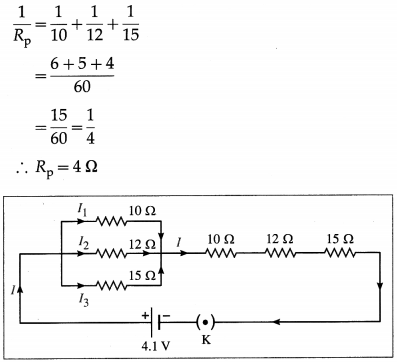For resistance Rp, 10 Ω, 12 Ω and 15 Ω connected in series, the equivalent resistance,
Rs = 4 + 10 + 12 + 15 = 41 Ω
Thus, the total resistance = Rs = 41 Ω
Now, V = IRs
∴ 4.1 = 1 × 41
∴ I = 0.1A
The total resistance and current through the circuit are 41 Ω and 0.1 A respectively.

Question 14.
A potentiometer wire has a length of 1.5 m and resistance of 10 Ω. It is connected in series with the cell of emf 4 Volt and internal resistance 5 Ω. Calculate the potential drop per centimeter of the wire.
Data : L = 1.5 m, R = 10 Ω, E = 4 V, r = 5 Ω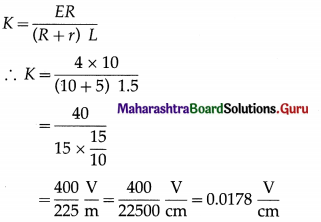The potential drop per centimeter of the wire is 0.0178 $$\frac{\mathrm{V}}{\mathrm{cm}}$$

Question 15.
When two cells of emfs. E1 and E2 are connected in series so as to assist each other, their balancing length on a potentiometer is found to be 2.7 m. When the cells are connected in series so as to oppose each other, the balancing length is found to be 0.3 m. Compare the emfs of the two cells.
Data : l1 = 2.7m (cells assisting),
l2 = 0.3 m (cells opposing)
E1 + E2 = Kl1 and E1 – E2 = Kl2
∴$$\frac{E_{1}+E_{2}}{E_{1}-E_{2}}=\frac{K l_{1}}{K l_{2}}$$
∴ $$\frac{E_{1}}{E_{2}}=\frac{l_{1}+l_{2}}{l_{1}-l_{2}}=\frac{2.7+0.3}{2.7-0.3}=\frac{3}{2.4}=\frac{30}{24}$$ = 1.25
The ratio of the emf’s of the two cells is 1.25.

Question 16.
The emf of a cell is balanced by a length of 120 cm of potentiometer wire. When the cell is shunted by a resistance of 10 Ω, the balancing length is reduced by 20 cm. Find the internal resistance of the cell.
Data :R = 10 Ω, l1 = 120 cm, l2 = 120 – 20 = 100 cm
r = R($$\frac{l_{1}-l_{2}}{l_{2}}$$)
= 10 ($$\frac{120-100}{100}$$)
= 2 Ω
The internal resistance of the cell is 2 Ω.Question 17.
A potential drop per unit length along a wire is 5 × 10-3 V/m. If the emf of a cell balances against length 216 cm of this potentiometer wire, find the emf of the cell.
Data: K = 5 × 10-3 $$\frac{\mathrm{V}}{\mathrm{m}}$$, L = 216 cm = 216 × 10-2 m
E = KL
∴ E = 5 × 10-3 × 216 × 10-2
= 1080 × 10-5
= 0.01080V
The emf of the cell is 0.01080 volt.
(Note: For K = 0.5 V/m, we get, E = 1.08V (reasonable value)]

Question 18.
The resistance of a potentiometer wire is 8 Ω and its length is 8 m. A resistance box and a 2 V battery are connected in series with it. What should be the resistance in the box, if it is desired to have a potential drop of 1 µV/mm?
Data: R = 8 Ω, L = 8 m, E = 2 V, K = 1 µV/mm
= 1 × $$\frac{10^{-6} \mathrm{~V}}{10^{-3} \mathrm{~m}}$$ = 10-3 $$\frac{\mathrm{V}}{\mathrm{m}}$$
K = $$\frac{V}{L}=\frac{E R}{\left(R+R_{\mathrm{B}}\right) L^{\prime}}$$ where RB is the resistance in the box.
∴ 10-3 = $$\frac{2 \times 8}{\left(8+R_{B}\right) 8}$$
∴ 8 + RB = $$\frac{2}{10^{-3}}$$
= 2 × 103
∴ RB = 2000 – 8
= 1992 Ω

Question 19.
Find the equivalent resistance between the terminals F and B in the network shown in the figure below given that the resistance of each resistor is 10 ohm.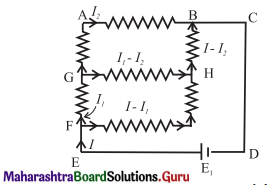Applying Kirchhoff’s voltage law to loop FGHF, we get,
– 10I1 – 10(I1 – I2) + 10(I – I1) + 10(I – I1) = 0
∴ – 10I1 – 10I1 + 10I2 + 10I – 10I1 + 10I – 10I1 = 0
∴ 201 – 40I1 + 10I2 = 0
∴ 2I – 4I1 + I2 = 0 …………….. (1)
Applying Kirchhoff’s voltage law to loop GABHG, we get,
– 10I2 – 10I2 + 10(I – I2) + 10(I1 – I2) = 0
∴ – 20I2 + 10I – 10I2 + 10I1 – 10I2 = 0
∴ 10I + 10I1 – 40I2 = 0 .
∴ I + I1 – 4I2 = 0 ……………… (2)
Applying Kirchhoff’s voltage law to loop EFHBCDE, we get,
– 10(I – I1) – 10(I – I1) – 10(I – I2) + E = 0
∴ -10I + 10I1 – 10I + 10I1 – 10I + 10I2 + E = 0
∴E = 30I – 20I1 – 10I2 ………….. (3)
From Eq. (1), we get, I2 = 4I1 – 2I …………. (4)
From Eqs. (2) and (4), we get,
I + I1 – 4(4I1 – 2I) = 0
∴ I + I1 – 16I1 + 8I = 0 .
∴ 9I = 15I1 ∴ I1 = $$\frac{9}{15}$$I = $$\frac{3}{5}$$I …………. (5)
From Eqs. (4) and (5), we get,
I2 = 4($$\frac{3}{5}$$I) – 2I = $$\frac{12}{5}$$I – 2I
= $$\frac{12 I-10 I}{5}$$ = $$\frac{2}{5}$$ I
From Eqs. (3), (5) and (6), we get
E = 30I – 20($$\frac{3}{5}$$ I) – 10($$\frac{2}{5}$$ I)
= 30I – 12I – 4I = 30I – 16I
∴ E = 14I
If R is the equivalent resistance between E and C,
E = RI
∴ R = 14 ΩQuestion 20.
A voltmeter has a resistance of 100 Ω. What will be its reading when it is connected across a cell of emf 2 V and internal resistance 20 Ω?
Data: E = 2V, r = 20 Ω, R = 100 Ω
The voltmeter reading, V = IR
V = ($$\frac{2}{100+20}$$)100 = $$\frac{200}{120}=\frac{10}{6}$$ = 1.667 V.

12th Physics Digest Chapter 9 Current Electricity Intext Questions and Answers

Observe and Discuss (Textbook Page No. 220)

Question 1.
Post Office Box
A post office box (PO Box) is a practical form of Wheatstone bridge as shown in the figure.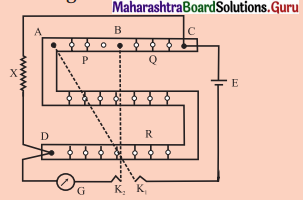It consists of three arms P, Q and R. The resistances in these three arms are adjustable. The two ratio arms P and Q contain resistances of 10 ohm, 100 ohm and 1000 ohm each. The third arm R contains resistances from 1 ohm to 5000 ohm. The unknown resistance X (usually, in the form of a wire) forms the fourth arm of the Wheatstone’s bridge. There are two tap keys K1 and K2 .
ρ = $$\frac{X \pi r^{2}}{L}$$